# High frequency Helmholtz coils generate magnetic fields

KC Yang

EDN

High frequency Helmholtz coils are often used to generate uniform but time varying high frequency magnetic fields for a number of applications such as magnetic field susceptibility, calibration, and scientific experiment. A high frequency Helmholtz coil (Ref. 1) driver is needed to generate the required magnetic field. Because the magnetic field density is proportional to electrical current, to generate high magnetic field, high current is needed. However, at high frequency the coils impedance is also became high impedance.

For a given driver voltage amplitude, the coil current is inversely proportional to the coil impedance. Therefore the two opposing factors that affect magnetic field are current and frequency. Achieving high frequency magnetic field is very difficult. This article discussed three techniques to produce high magnetic field for high frequency Helmholtz coils.

## High Frequency Helmholtz Coils Basic

Helmholtz coils, named after the German physicist Hermann von Helmholtz, is consisted of two identical electromagnetic coils place in parallel and aligned their centers in the same axis like a mirror image as shown in Figure 1. When electrical current pass through the high frequency Helmholtz coils in the same direction, it creates a highly uniform magnetic field in a 3-dimension volume of space inside the coils. These Helmholtz coils are often used for cancel background (earth’s) magnetic field, measurements and calibration, and magnetic field for susceptibility testing of electronic equipment.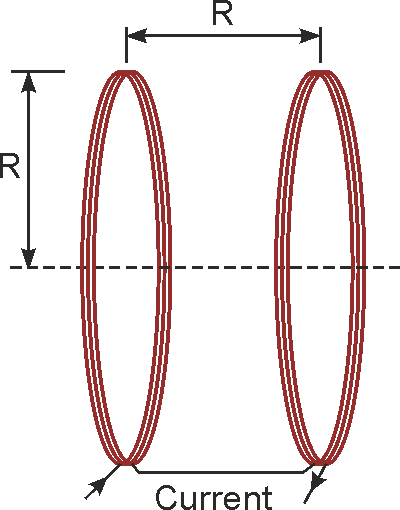Figure 1. One-axis high frequency Helmholtz coils is consisted a pair of coils with radius R and separated by a distance equal to R.

## Helmholtz Coils Design and Construction

High frequency Helmholtz coils are constructed by two coils. Because the two magnetic coils are designed to be identical, uniform magnetic field is achieved when the coil radius is equal to the separation distance. The two coils are connected in series such that identical current feeding both of them creates two identical magnetic fields. The two added fields achieved uniform magnetic field in a cylindrical volume of space in the center between the two parallel coils.

This cylindrical-shaped volume space uniform field is approximately equal to 25% of coil radius (R) and a length equal to 50% of the spacing between the two coils. High frequency Helmholtz coils are available in 1, 2, or 3 axes. Multiple axis magnetic coils generate magnetic fields in any direction in the three dimensions space inside the Helmholtz pair. The most common high frequency Helmholtz coils are circular. Square Helmholtz coils are also commonly available.

## Helmholtz Coils Magnetic Field Calculation

Each Helmholtz coil is constructed by loops of electrical (copper) wires. When electrical current is passing through it, magnetic field is generated. The magnetic field density is proportional to current. The Helmholtz coils magnetic field equation is given below.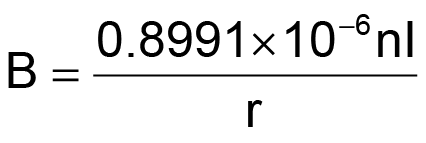(1)

where

B – field in Tesla,
n – number of turns in a coil,
I – current in amperes,
r – coil radius in meters.

From Equation 1, smaller radius coil generates higher magnetic field density. Also the more number of turns in each coil the stronger the magnetic field.

## High Frequency Helmholtz Coils Model

Helmholtz magnetic field is generated either using AC or DC current. Most Helmholtz coils applications are static (constant) magnetic field using DC current. Some applications such scientific experiments required non-static magnetic fields at high frequency (kHz to MHz). This article is mainly discussing high-frequency Helmholtz coils.

A pair of high frequency coils can be model as shown in Figure 2. Each coil can be modeled as a parasitic resistor in series with an ideal inductor. The parasitic resistor resistance is generally small. For most high frequency Helmholtz coils application where the testing frequency is well below the self-resonant frequency, this model is sufficient.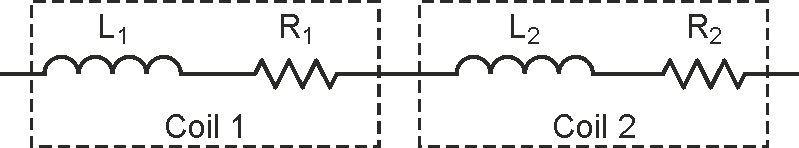Figure 2. Circuit model of two Helmholtz coils connected in series.

If the Helmholtz coil operating frequency is close enough to its self-resonant frequency, the circuit model must also include its parasitic capacitances (CP1 and CP2). The parasitic capacitors are parallel to each inductor and resistor in series as shown in Figure 3.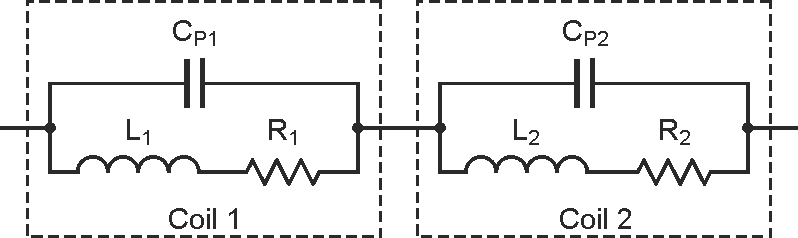Figure 3. High-frequency Helmholtz coils are modeled as two LCR circuits connected in series.

The parasitic capacitance and inductance formed a self-resonant frequency. Although the coils are designed to be as closely match as possible, but some small variations between them are expected. Each coil has its own series resistance and parasitic capacitance.

## High Frequency Helmholtz Coils Connections

High frequency Helmholtz coils may be connected in series (Figure 2) or in parallel as shown in Figure 4. Series connection allows the same electrical current flow through the two magnetic coils. Generally series connection enables the highest current and thus highest magnetic field. However, because two coils are in series, the total impedance is also double. Higher impedance may require higher driver amplifier voltage. If used resonant techniques described below, the impedance is reduced.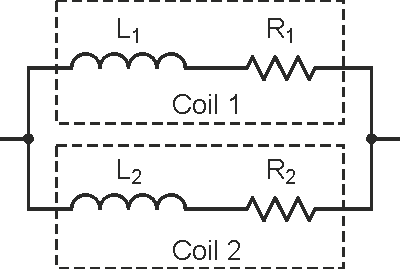Figure 4. Helmholtz coils are connected in parallel.

The advantage of parallel Helmholtz coil connection is lower impedance. In fact the impedance is cut in half, but the current is also cut in half (current is split into two). Thus lower the magnetic field. Parallel connection is acceptable if the required magnetic field density is achieved at half the current and low impedance is required such as the case of low-voltage amplifier driver. More details on Helmholtz coil impedance below in the Direct Drive Method section.

## Driving High Frequency Helmholtz Coils

There are three ways to produce high frequency AC magnetic field. The first method is direct drive method. This method is the simplest way to produce magnetic field for testing. It is very easy to vary the frequency and magnetic field under test. The second method is series-resonant method. This method is a powerful way to produce high magnetic field and very high frequency in the order hundreds kHz or even MHz. The third way is using a new current-amplified resonant method. This method generates the highest magnetic field density. The below sections will describe each method.

## Direct Drive Method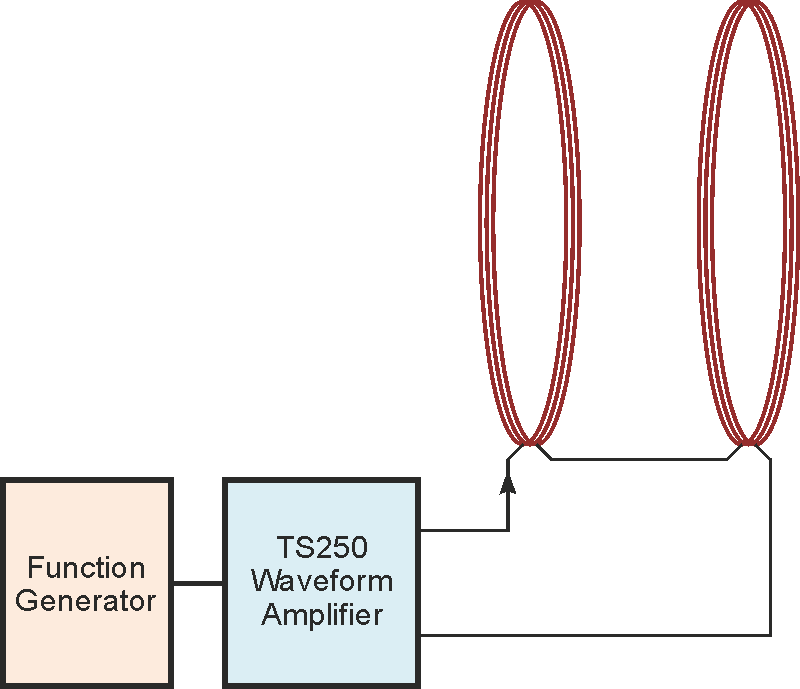Figure 5. TS250 Waveform Amplifier drives a pair of Helmholtz coils.

If the experiment is low frequency or the coils are low inductance or both, the Helmholtz coils may be driven directly using a waveform amplifier driver such as the TS250 Waveform Amplifier from Accel Instruments. Because of low frequency or low inductance, the coil’s impedance is low enough it can be driven by an amplifier directly as shown in Figure 5 and Figure 6.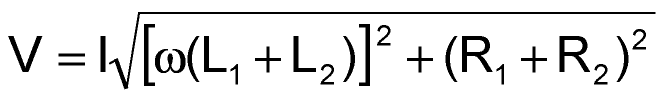(2)

where

I is the peak current,
w is the angular frequency, w = 2pf,
L1 + L2 are the total inductance,
R1 + R2 are the total resistance.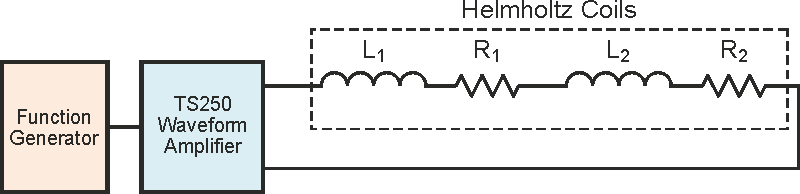Figure 6. Circuit representation of a Waveform Amplifier directly drives a pair of Helmholtz coils connected in series.

Use the Equation-1 to calculate the coil current for a desired magnetic field. Then use Equation-2 to calculate the maximum voltage is needed. Note the small parasitic resistance is ignored. The maximum voltage is when the current and frequency are both at maximum. The next step is to drive the Helmholtz coils with a high-current and high-frequency amplifier driver such as the TS250 function generator amplifier.

## Series Resonant Method

If the generated magnetic field is high frequency, Helmholtz coils impedance increases with frequency (Z = jwL). At high frequency the coil impedance is very high such that high voltage is needed to drive high current through the coil. For example, at 200 kHz the impedance of a 2 mH coil will be 2512 ohm. If you drive the coil with a 40 V for example, you would get about 16 mA (40 V/2512 ohm = 16 mA). For most applications, this is not enough current to produce enough magnetic field. For high magnetic field applications, higher current through the coil is desired. To drive a 2 A high-current through the coil, 5024 V is needed! It is difficult to generate 5 kV at 200 kHz.

To achieve high-current and high-frequency electromagnetic field, series resonant technique can be recommended.

To operate the high frequency Helmholtz coils in resonance mode, a series capacitor is added as shown in Figure 7. The series capacitor impedance has an opposite polarity than the inductor. Thus the capacitor is acting as an impedance cancellation device. It reduces the total impedance. At resonance the capacitor reactance (imaginary portion of the impedance) is completely cancels the inductor reactance. That is the inductor and capacitor reactance are equal magnitude but opposite polarity.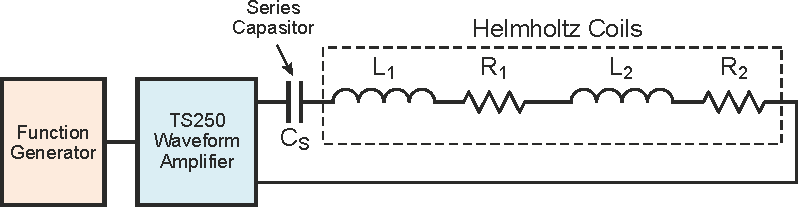Figure 7. Waveform amplifier drives high current through the Helmholtz coils at resonance.

Only the inductor’s parasitic resistance remained. With only resistance remained, the function generator amplifier (TS250) can drive high current through the Helmholtz coils (LCR circuit) even at high frequency. This method enables the signal amplifier to drive high current through the high frequency coils, but it can only operate at a very narrow frequency range near resonance. The disadvantage of resonant technique is that you need to change the capacitance when you change the frequency.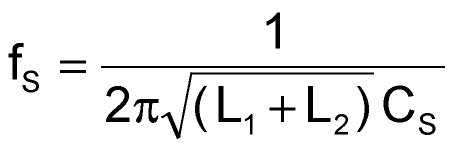(3)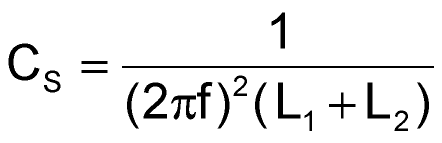(4)

The series-resonant frequency of the Helmholtz coils is given in Equation-3. The series capacitance, CS, is calculated using Equation-4. The voltage across the series capacitor is given in Equation-2 above. At high frequency and high current, the voltage could be in the thousands volts. For example, at 200 kHz and 1 A current though a 2 mH high frequency Helmholtz coils, the voltage across the capacitor is 2512 V! The capacitor must be rated for at least that voltage.

## Caution: Potential Electrical Shock

High-current Helmholtz (electromagnetic) coils discussed above can store enough energy to become an electrical shock hazard. Make sure all electrical connections are insulated with high-voltage insulators. Wires must be rated for voltages rating discussed above. Always disable the waveform amplifier output before connecting or disconnecting the coil and capacitor.

## Current-Amplified Resonant Method

Another resonant that is even more powerful than the series resonant is called the current-amplifier resonant. This newly discovered resonant can boost the Helmholtz coils current by a factor of two. That is the coil current is twice the source amplifier driver current. Hence the resonant is magnifying current and the magnetic field. Detail information of this newly discovered resonant can be found in the application note High Frequency Magnetic Field Generator (Ref. 2).

Figure 8 shows the high frequency Helmholtz coils connection using the current-amplified resonant. Two equal value capacitors are needed. One capacitor is connected in series with the coils, similar to the series resonant discussed above. A second resonant capacitor is connected in parallel with the two coils. This parallel capacitor is similar to the parasitic capacitors discussed above in the high frequency Helmholtz coils circuit models.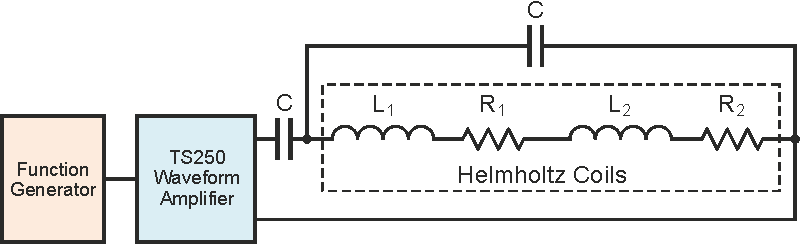Figure 8. High frequency Helmholtz field using Current-Amplified Resonant to doubles the magnetic field.

The resonant frequency is expressed in Equation-6. The two capacitor values are calculated in Equation-8. At resonant, the Helmholtz coils impedance is resistive and 4 times the coils parasitic resistance. It is desirable to design low resistance coils when used in the current-amplified resonant. Also keep in mind the magnetic coil’s AC resistance is higher than DC due to the skin-effect.(5)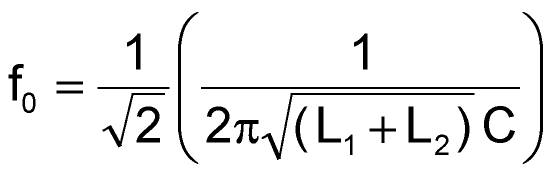(6)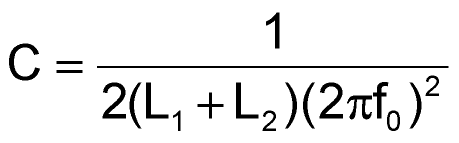(7)

## Conclusion

Three methods for driving high frequency Helmholtz coils are discussed in this article. The direct-drive method is the simplest, but generally for low frequency or low inductance. Driving Helmholtz coils using the series resonant method generates high current and high frequency magnetic field. The novel current-amplified resonant produces even higher magnetic field even at high frequency.

EDN